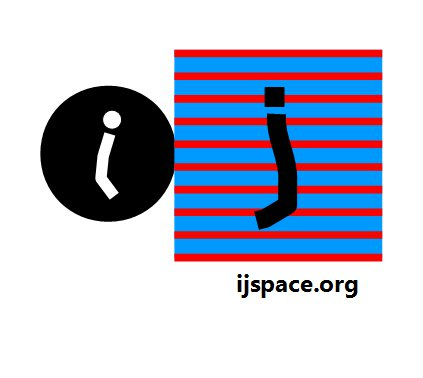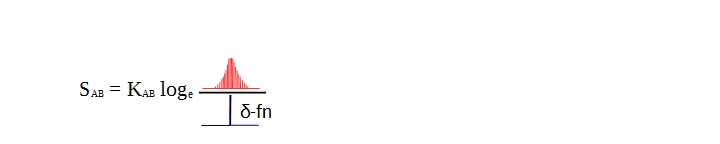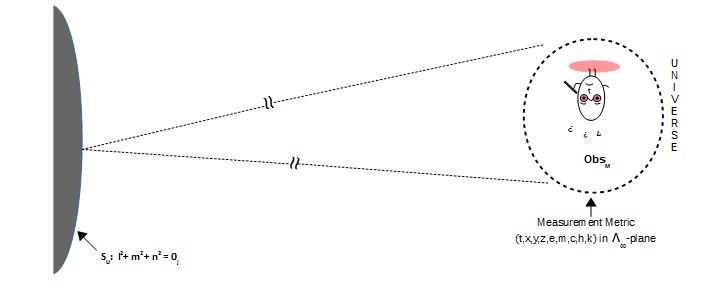# Home

 In a Nutshell-2016 28th December 2016 “I confess that once when I was very young I confused the Leeds Mercury with the Western Morning News. But a Times leader is entirely distinctive, and these words could have been taken from nothing else. The address, you observe, is is printed in rough characters. But The Times is a paper which is seldom found in any but in the hands but those of the highly educated. We may take it, therefore, that the letter was composed by an educated man who wished to pose as an uneducated one.” -Sherlock Holmes in The Hound of Baskervilles. "In effect, Shannon single handedly  accelerated the rate of scientific progress, and it is entirely possible that, without his contribution, we would still be treating  information as if it were some ill-defined vital fluid." - James Stone in his excellent "Information Theory: A Tutorial Introduction". The book by Stone on Bayesian Analysis is very highly recommended. The year 2016 had been an interesting one in terms of the discovery of new concepts and maturing the existing ones. Some of which we reported here, others such as Quantum Bayesianism and the fascinating ideas behind the development of Quantum Computing, we have not. At least not yet.     The Bayes Probability theory is one of the most remarkable and sophisticated piece of work. In another dimension of our mundane existence, it is also used for solving important problems such as the anomaly detection and the parameter classification in very high speed communication networks. However it is the usefulness of Bayesian Probability in eliminating the unnecessary noise surrounding the Quantum Mechanics and its perceived (mis)interpretation that the universe is random or probabilistic in nature, which is compelling1.     Similarly another powerful idea which will guide the progress in foreseeable feature, is the idea of Reversible Computing also known with its rather alluring name Quantum Computing. While a killer application for quantum computing is far from reality, the notion that knowing the nature of gate and looking at the output(s) one can determine the input(s), is fetching2. It is a step towards developing 'intuition' in our observations. Essentially we move from complex to elementary.     If we think carefully, in both ideas i.e. the Bayesian Probability and the Reversible Computing, the observer plays a central role. More knowledgeable the observer, more accurate is the deduction or the estimate of the initial state by the observer3. This simple principle also forms the core of the j-space or discrete measurement space, work on which is being reported on this site.     We will discuss these ideas later on. Right now we return the original premise of this particular blog titled Nutshell-2016. We summarize the work we reported during the the year 2016 as follows: 1. We stated the problem of measurement for an arbitrary path AB. We discussed the correlation between the probability distributions and the observer capabilities. Crucially the problem of measurement was independent of the observer's measurement-metric i.e. all the observers (electron, photons, protons, neutrons, atoms, molecules, quarks, planets, stars, black-holes, galaxies, human, ents, vulcans etc.) measure the same path AB, but their measurements will yield different results based on their respective capabilities. These results are essentially the subsets of the superset containing all the information contained in the path AB.    During this discussion we also introduced the necessity of the quanta. Please note that the need for the quanta was purely phenomenological without the consideration of the quanta associated with radiation. 2. We next discussed the definition of a 'paradox' in terms of the Axiom of Foundation. We then discussed the difference between the "finiteness" and the "quantum" using  VT-symmetry,  Hamilton's Anharmonic Coordinates, Pauli's Spin Matrices and 4π-symmetry. 3. We continued with the discussion on what it meant by "duality" in j-space, rather than launching straight into conventional wave-particle duality. We introduced an unit of efficiency "ĥ". We showed that the resources needed by the low efficiency observer to travel path C-D will be an integral multiple of  ĥ. We further discussed "discreteness" which was defined by the zero-entropy path. The conventional wave description was associated with the minimum-entropy or finite-entropy description of the path AB. The discussion so far, was needed to understand the celebrated De Broglie relationship in j-space. 4. Next we developed the relationship between the information content extracted by an observer from a path being measured in j-space as follows:Above equation is phenomenological equivalent to De Broglie equation, in j-space. To derive this relation we used the following essential characteristics of any arbitrary observer in a j-space:         1. Ability to extract information represented by variables "v/c" and ƛ,         2. Ability to correct its course represented by the variable ƛ,         3. Ability to stay the course represented by the variable ν. We note that a path being measured in j-space, is equivalent to the interval [0,1]. 5. We visualized Entanglement in terms of "Skeleton" structures4. The "Skeleton" structures in this case corresponded to Hamilton's Anharmonic Coordinates i.e. unit-point U, ΔOXY, unit-point sphere SU, and Λ∞-plane. The basic idea is shown below:The macroscopic observer would be able to determine only the structures shown on the left, which represents the classical picture. However it would not be able to measure the relationship between Λ∞-planes corresponding these structures. For the macroscopic observer the objects A and B are entangled.     The observer Obsi (v/c >> 1) our own Maxwell demon in j-space, would be accurately measuring this relationship and hence based on the measurements made on object-A, Obsi could predict the behavior of the object-B.  Which also meant that the Locality Condition restricting measurement of Λ∞-planes, has to be eliminated. (This is where j-space description differs from QBism). If we could not determine Λ∞-planes accurately, then the probability based Quantum Mechanical description of physical reality would be needed to make estimates. 6. One of the critical quantity in the budget allocated to a universe, is the minimum resource required to measure a given path, also known as "Action" S. The action S and Hamiltonian H are connected by Hamilton-Jacobi equation, whose reduced form leads to Schrödinger equation. The action SAB for the path AB is related to the wave-function ψ as SAB = KAB logeψ. The relationship between action SAB and the wave-function ψ can be shown figuratively as following:To sum up, the wave-function represents a small fraction of the information-sample as measured by ObsM from an infinitely large sample population. The estimation is represented by a probability distribution hence the stochastic nature of ψ. The minimum resource required to travel path AB is ĥ.      Furthermore above diagram represents only 1 degree of freedom in observer's measurement space. The actual initial state being measured, may require a description with infinite degrees of freedom and that is where Bayesian theory and Conditional Probabilities are likely to play an important role. 7. One of the objectives is to measure or estimate the resources available at the initial state of the universe or equivalently at the starting point A of the path (A →B).  The constant KAB plays an important role in making this assessment. For a macroscopic observer ObsM, the constant KAB will represent different physical constants corresponding to Action S,  for different paths being measured. Different paths imply different information spaces and subsequently different nature of corresponding disorders ΩM's. The quantity ΩM represents macro-states in thermodynamic system and quantum-states for a quantum system.     For the macroscopic observer (v/c <<1), for measurements in a lower information space (v/c < 1) KAB will be the Boltzmann physical constant kB (J.K-1), whereas for measurements in a higher information space (v/c ~ 1) KAB will be the Planck universal constant h (J.s). We will discuss the significance of the constant KAB in the information space later on.     So can we say that the Quantum Mechanics is a universal theory? It is not. The objects in universe do not obey the laws of probability but humans use these laws in a complex calculation space, to make estimates as accurately as possible to match the measurements made in the observer's measurement space. In the following figure Quantum Mechanic explains information within the measurement metric called UNIVERSE, but not the unit-point sphere SU or the structure within.As humans evolve biologically, the estimates will become better and so will the understanding of this universe. Furthermore throughout the evolution, Bayesian probability probably is the best option to make precise estimates. _______________________ 1. We would like to thank Professor C. A. Fuchs for providing us with some of his writings on QBism. 2. An example of reversible computing is NOT gate in Classical Computing. If we knew the O/P state and the nature of gate, the I/P state could be predicted. For example for O/P of NOT gate equal to '1' we can deduce the I/P as '0' and vice versa. However we must also keep in mind that NOT gate is not an universal gate i.e all the problems which could be solved by Classical Computing, can not be solved by NOT gate and its combinations alone. In classical computing 'NOR' and 'NAND' gates are universal gates. 3. With more and more information available to the observer, the observer's estimate of the initial state moves from an arbitrary probability distribution towards δ-function or PE1 measurement. 4.The "Skeleton" structures are determined based on the description provided by Obsc (v/c ~ 1) and therefore the system based on "Wrap" parameters i.e. parameters corresponding to the macroscopic observer ObsM (v/c << 1) must follow the system based on "Skeleton" structures. ("Wrap" around "Skeleton" to form a shape or an observation.) Previous Blogs: Quanta-II Quanta-I EPR Paradox-II EPR Paradox-IBest viewed with Internet Explorer